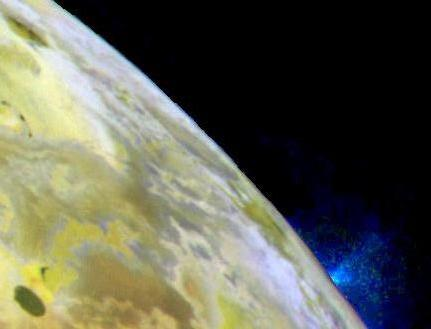October 12, 2008

This Week’s Finds in Mathematical Physics (Week 270)

Posted by John BaezIn week270 of This Week’s Finds, see lava flowing on Jupiter’s moon Io.Hear about Greg Egan’s brand new novel, Incandescence. Then - see talks about my favorite three numbers. Read about the Mathieu group M12. Learn how each regular polytope with 5-fold rotational symmetry is secretly linked to a lattice in a space of twice as many dimensions. And see a simple but surprising property of the number 12.

Posted at October 12, 2008 1:33 AM UTC

TrackBack URL for this Entry:   https://golem.ph.utexas.edu/cgi-bin/MT-3.0/dxy-tb.fcgi/1815

Connes-Egan; Re: This Week’s Finds in Mathematical Physics (Week 270)

Week 270 = 2 * 3^3 * 5 is delightful. Even through the stench of brimstone…

Suppose that physical space is actually a noncommutative geometry. What primitive aliens in an exotic environment could figure that out? I await the Connes-Egan collaboration…

Posted by: Jonathan Vos Post on October 12, 2008 7:28 AM | Permalink | Reply to this

Re: This Week’s Finds in Mathematical Physics (Week 270)

I’m confused by your description of the (5,6,12) Steiner system. If I apply the transformation z -> 1/z to the block (0,1,3,4,5,9), I get (infty,1,4,3,9,5), which has a 5 element overlap with the original block. My guess is that I am supposed to only apply transformations z -> (az+b)/(cz+d) where ad-bc=1. Is this right?

Posted by: David Speyer on October 12, 2008 10:08 PM | Permalink | Reply to this

Re: This Week’s Finds in Mathematical Physics (Week 270)

You’re right. Fixed.

My dejargonization program hit a bug while processing the term ‘PSL(2,11)’.

Posted by: John Baez on October 12, 2008 10:44 PM | Permalink | Reply to this

Re: This Week’s Finds in Mathematical Physics (Week 270)

John B. wrote:

People often say that Penrose tilings arise from lattices in 4d space. Maybe I’m finally starting to understand how! The $A_4$ lattice has a bunch of 4-simplices in it — but when we project these onto the plane correctly, they give pentagrams. I’d be very happy if this were the key.

It is the key! In fact Greg Egan has an applet based on this idea! It draws quasiperiodic tilings with 5-fold symmetry formed by slicing the $A_4$ lattice with a cleverly chosen plane.

Even better, it draws quasiperiodic tilings with $n$-fold symmetry formed by slicing the $A_{n-1}$ lattice with a cleverly chosen plane… when $n$ is odd. For $n$ even, it uses a slightly different trick… but I think we’re seeing a general relation between the noncrystallographic Coxeter group

n
o---o

and $A_{n-1}$, which generalizes the $n = 5$ case I talked about.

Even better, we can indeed get 3d quasicrystals with dodecahedral symmetry from slicing a $D_6$ lattice in 6 dimensions…

… and we can get 4d quasicrystals with the symmetry of the 120-cell from slicing an $E_8$ lattice in 8 dimensions!

• J. F. Sadoc and R. Mosseri, The $E_8$ lattice and quasicrystals, J. Non-crystalline Solids 153,154 (1993) 247–252.
• R. V. Moody and J. Patera, Quasicrystals and icosians, J. Phys. A. 26 (1993), 2829–2853.

The latter paper is tremendously fun; I haven’t looked at the former yet.

I will write more about this later. I was always scared of quasicrystals, but now I’m not, because I see how they connect to things I like!

Posted by: John Baez on October 13, 2008 4:10 PM | Permalink | Reply to this

Post a New Comment